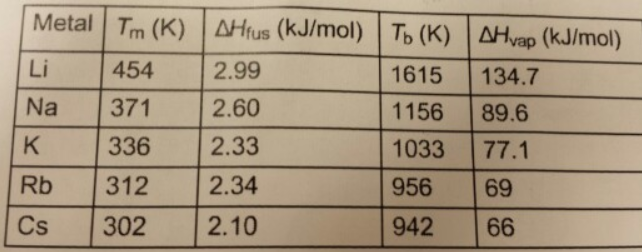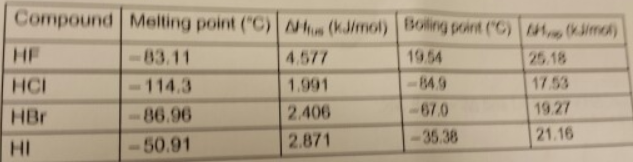# Problem: 1. Using the following data,Calculate ∆Sfus and ∆Svap for Na.ΔSfus = J•K-1•mol-1ΔSvap = J•K-1•mol-12. Using the following data,calculate the ΔSfus and ΔSvap for HBr.ΔSfus = J/(K•mol)ΔSvap = J/(K•mol)Determine the entropy change when 6.60 mol of HBr(l) boils at atmospheric pressure.ΔS = J/K

###### FREE Expert Solution

$\overline{){\mathbf{∆}}{\mathbf{S}}{\mathbf{=}}\frac{\mathbf{∆}\mathbf{H}}{\mathbf{T}}}$

Calculate ∆Sfus and ∆Svap for Na.

$\mathbf{∆}{\mathbf{S}}_{\mathbf{fus}}\mathbf{=}\frac{\mathbf{∆}{\mathbf{H}}_{\mathbf{fus}}}{{\mathbf{T}}_{\mathbf{m}}}$

97% (396 ratings)###### Problem Details

1. Using the following data,Calculate ∆Sfus and ∆Svap for Na.

ΔSfus = J•K-1•mol-1

ΔSvap = J•K-1•mol-1

2. Using the following data,calculate the ΔSfus and ΔSvap for HBr.

ΔSfus = J/(K•mol)

ΔSvap = J/(K•mol)

Determine the entropy change when 6.60 mol of HBr(l) boils at atmospheric pressure.

ΔS = J/K